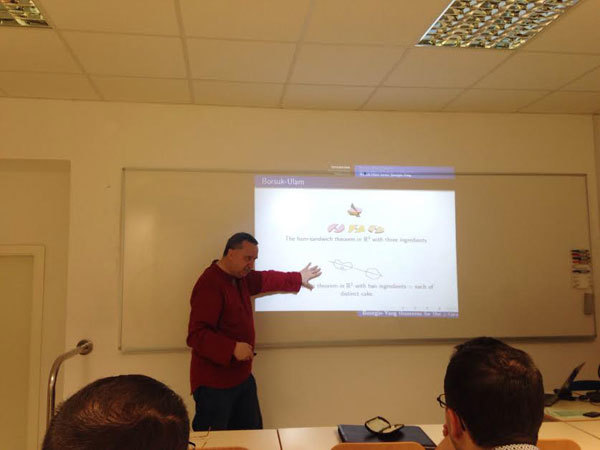Univerza na Primorskem Fakulteta za matematiko, naravoslovje in informacijske tehnologije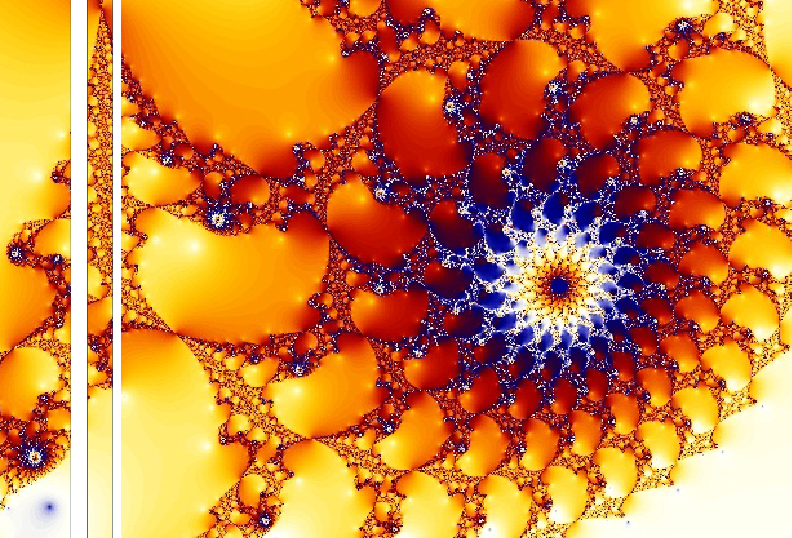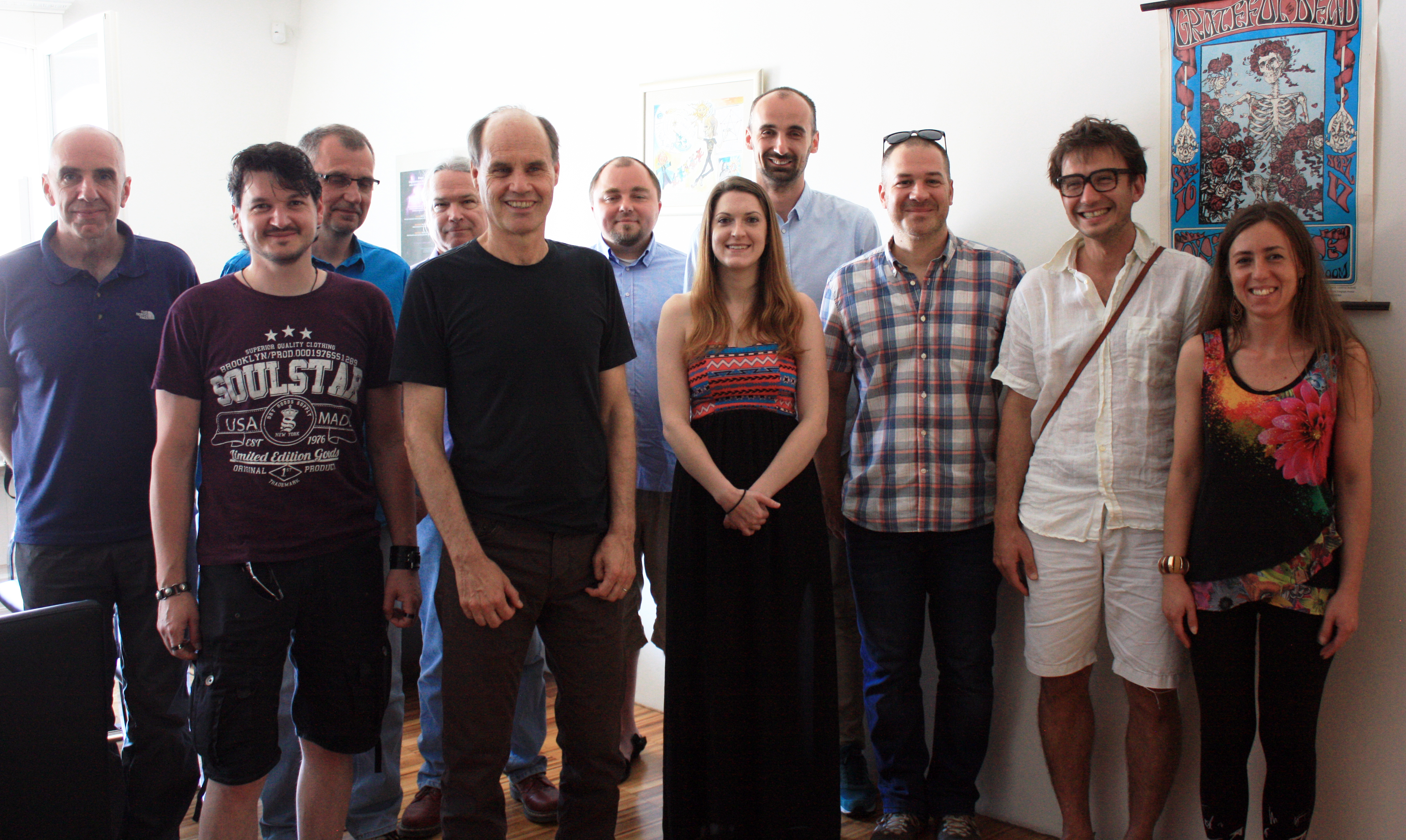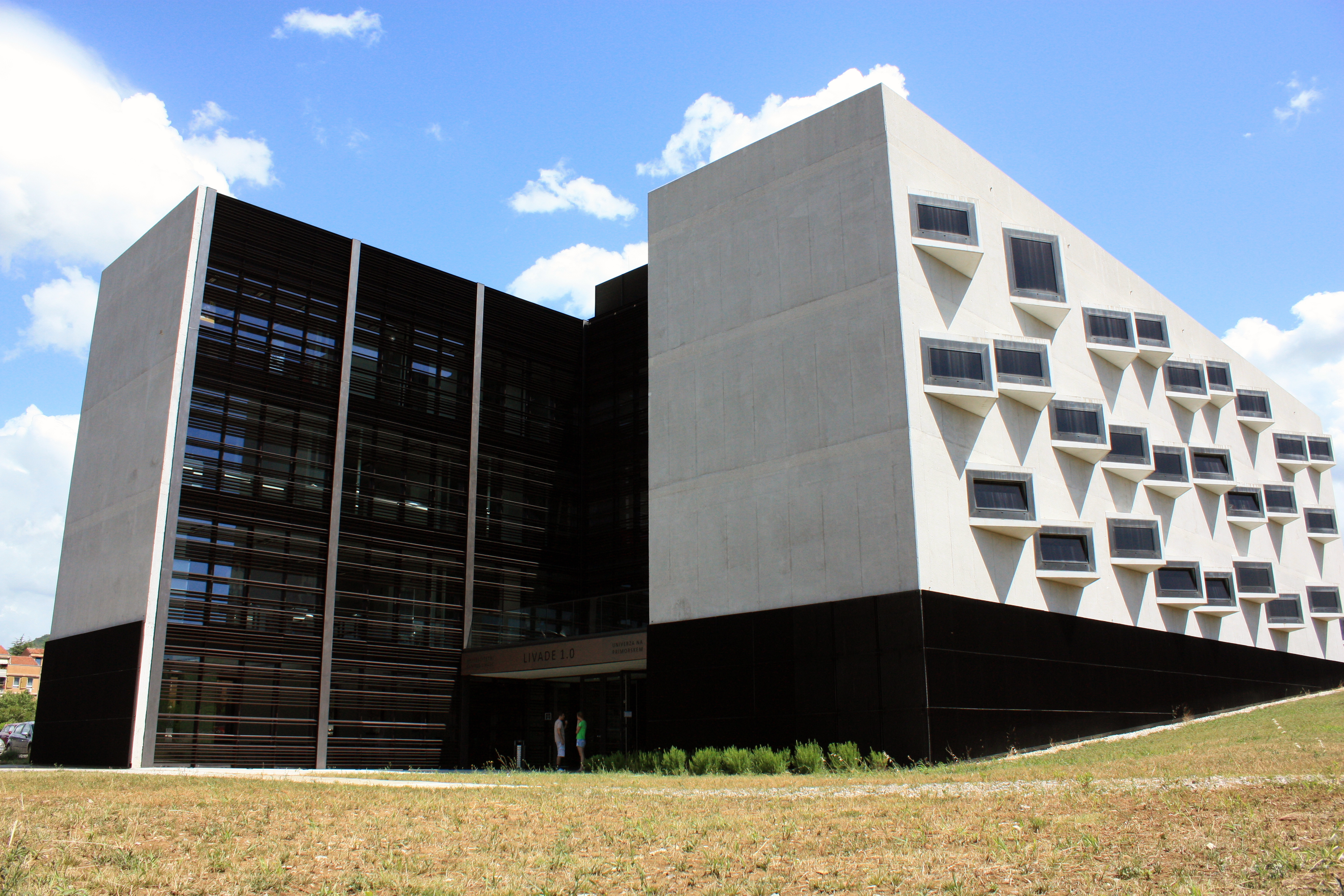SI | EN# Raziskovalni matematični seminar - Arhiv

 2023 2022 2021 2020 2019 2018 2017 2016 2015 2014 2013 2012 2011 2010
 1 2 3 4 5 6 7 8 9 10 11 12
Datum in ura / Date and time: 6.6.16
(10:00-11:00)
Predavalnica / Location: FAMNIT-Muzejski1
Predavatelj / Lecturer: Ana Zalokar (UP IAM & UP FAMNIT)
Naslov / Title: Optimal switching among different hedging strategies
Vsebina / Abstract:

The motivation for our research problem comes from hedging reserves in life insurance policies with guarantees. Since some supervisory authority advise to take a hedging strategy, where the number of units of a risky asset is determined to always be one, one should consider if there is some other hedging strategy, which would be at some point better to use. If there is such, when would be the optimal time to switch from one to another? We will look at some simulations that confirms our assumption of existing such hedging strategy and we will look when would be optimal to switch in a general mathematical case. This is joint work with Mihael Perman.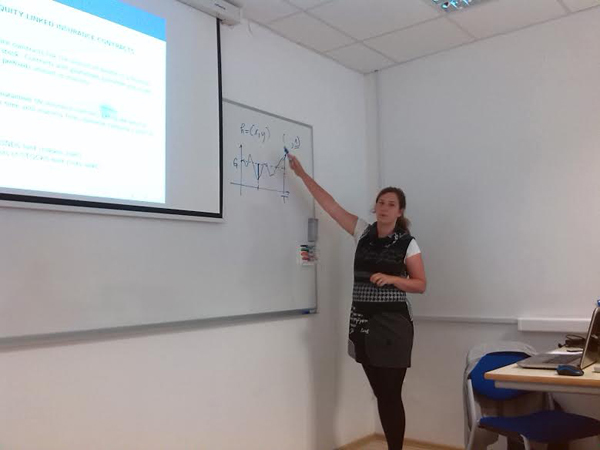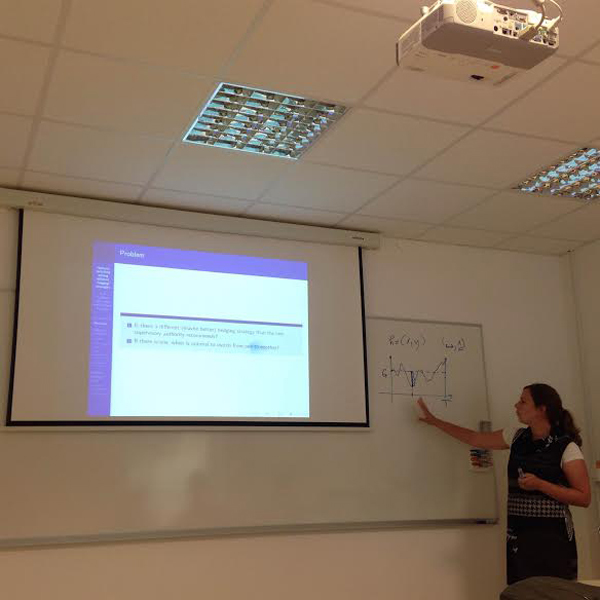Datum in ura / Date and time: 6.6.16
(11:00-12:00)
Predavalnica / Location: FAMNIT-Muzejski1
Predavatelj / Lecturer: Samir Hodžić (UP IAM & UP FAMNIT)
Naslov / Title: Full characterization of generalized bent functions
Vsebina / Abstract:

In difference to many recent articles that deal with generalized bent (gbent) functions $f:\mathbb{Z}_2^n \rightarrow \mathbb{Z}_q$ for certain small valued $q\in \{4,8,16 \}$, we give a complete description of these functions for both $n$ even and odd and for any $q=2^k$ in terms of both the necessary and sufficient conditions their component functions need to satisfy. This enables us to completely characterize gbent functions as algebraic objects, namely as affine spaces of bent or semi-bent functions with interesting additional properties, which we in detail describe. We also specify the dual and the Gray image of gbent functions for $q=2^k$. We discuss the subclass of gbent functions which corresponds to relative difference sets, which we call $\Z_q$-bent functions, and point out that they correspond to a class of vectorial bent functions. The property of being $\mathbb{Z}_q$-bent is much stronger than the standard concept of a gbent function.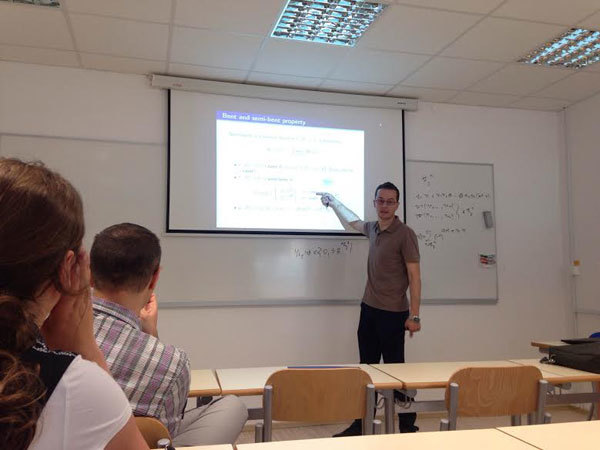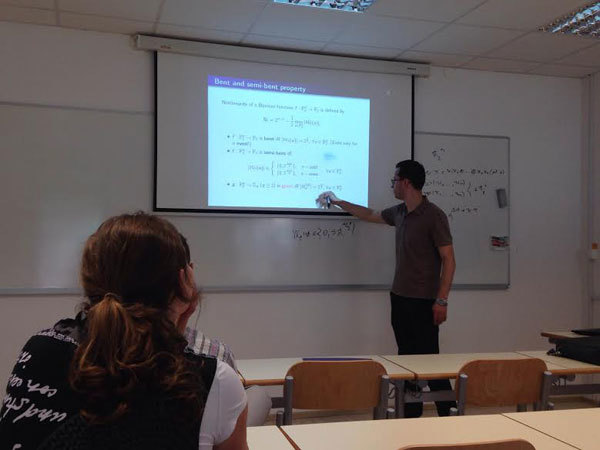Datum in ura / Date and time: 9.6.16
(10:00-11:00)
Predavalnica / Location: FAMNIT-Muzejski3
Predavatelj / Lecturer: Waclaw Marzantowicz (Adam Mickiewicz University, Poznań, Poland)
Naslov / Title: Bourgin-Yang theorems for the $p$-toral groups
Vsebina / Abstract:

In 1933 S. Ulam posed and K. Borsuk showed that if $n>m$ \newline then {\bf{it is impossible}} to map $f: S^n \to S^m$

\centerline{preserving symmetry: $f(-x)= -f(x)$ .}

Next in 1954-55, C. T. Yang, and D. Bourgin, showed that if $f: \mathbb{S}^n\to \mathbb{R}^{m+1}$ preserves this symmetry then

\centerline{$\dim f^{-1}(0) \geq n-m-1$.}

We will present versions of the latter for some other groups of symmetries and also discuss the case $n=\infty$

Let $V$ and $W$ be orthogonal representations of a compact Lie group $G$ with $V^G = W^G=\{0\}$.

Let $S(V )$ be the sphere of $V$ and $f:S(V ) \to W$ be a $G$-equivariant mapping.

We estimate the dimension of set $Z_f=f^{-1}\{0\}$ in terms of $\dim V$ and $\dim W$, if $G$ is the torus $\mathbb T^k$, the $p$-torus $\mathbb Z_p^k$, or the cyclic group $\mathbb Z_{p^k}$, $p$-prime.

Finally, we show that for any $p$-toral group: $\;e\hookrightarrow \mathbb{T}^k \hookrightarrow G \to \mathcal{P}\to e$, $P$ a finite $p$-group and a $G$-map $f:S(V) \to W$, with $\dim V=\infty$ and $\dim W<\infty$, we have $\dim Z_f= \infty$

Bourgin-Yang_Primorska.pdf